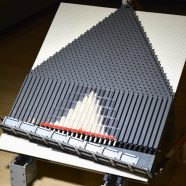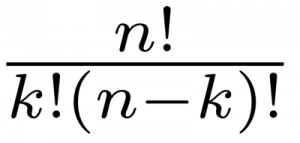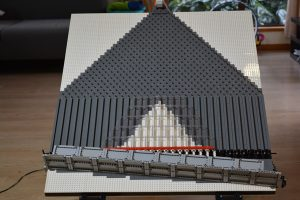# Quincunx LEGO GBC Module (Galton Board)

Posted by on Jul 2, 2016 in Featured, LEGO, Research | 5 commentsToday I would like to show you my latest GBC module, a Quincunx also known as a Galton Board, named after its inventor Sir Francis Galton who used it to demonstrate the central limit theorem in 1894. The balls are being transported up with a conveyer belt and a light sensor counts how many balls have passed. The balls then roll down the board and at each peg they can either bounce left or right. After the last peg the ball is caught in a repository. Once 100 balls made their way down, the gate opens and releases all the balls. Probably no GBC module could deal with 100 balls at a time, so I queued them up and deliver them one at a time.

The chances of the ball going left or right at each peg are even. So you might conclude that the balls should be evenly distributed amongst all repositories. But that is not the case and it takes a little bit of math to understand why.

Lets assume we only had three rows of pegs. That means that a ball would have to make only three decisions to either turn left or right. This means the number of total possibilities is 2x2x2=8. We can also write this shorter more generally as 2 to the power of n, where n is the number of rows on the board. We can list all eight possible paths for this board:

R0 R1 R2 R3
RRR 1
RRL 1
RLR 1
LRR 1
RLL 1
LRL 1
LLR 1
LLL 1
Probability 1/8 3/8 3/8 1/8

What we observe is that the sequence of decision does not matter. A ball that the goes the path LRR will end up in the same repository as the ball that went RRL.  We can add up the number of paths to a repository and divide it by the total number of possibilities to calculate the probability of a ball landing in a specific repository. We can even consider the index number of the repository as the number of right turns the ball took.

The chances for balls to end up in repository are unevenly distributed because a different number of paths lead to them. If we would let 1000 balls flow down this board then 125 are likely to end up in R0, 375 in R1 and R2 and 125 in R3. The reason for this is that there are more paths to R1 and R2 than to R0 and R3.

In math we like to generalize our results to any number of pegs, repositories and balls. First we need to find out how many different paths exist for a ball to end up in an arbitrary repository, which is equivalent to the number of right turns. The formula is this:Where n is the number of decision, which in our case is 3, and k is the number right turns. For a ball to get into R2 it needs two 2 right turns. This means that the number of paths to get there is :There are 3 ways and hence the probability of a ball to go to repository 2 is 3/8. This Quincunx GBC module has 32 repositories which means that the balls have to make 31 decisions to either turn left or right. Using the formula above we can calculate that the probability for each repository and show it in a graph. This graph is fundamentally a binominal distribution.The white in the repositories area is exactly this expected frequency distribution. This is where we expect the balls to fall. For a statistical test 100 balls is a rather small number and hence the distribution of the balls do slightly differ from the expected distribution.I hope you enjoyed this Quincunx GBC module and the math behind it.

1.Do you know where I can purchase such a board? Thank you.
Alan

2.I am not aware of any place where you can purchase this. I made it myself but I disassembled it by now. I could rebuild it, but that would take time and resources. Send me an email if you are interested.

3.How wonderful! I am interesting in buying that thing. Would you send an estimate to my adress? Best greetings from Vienna.

4.Hi,

I just send you an email.

/c

5.Dear sir,

Your Quincunx LEGO GBC Module looks great. I am also interested in acquiring a set. Can you help, please?

Phillip